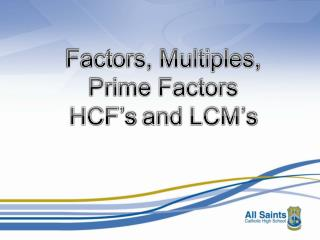DownloadDownload PresentationFactors, Multiples, Prime Factors HCF’s and LCM’s

# Factors, Multiples, Prime Factors HCF’s and LCM’s

Download Presentation## Factors, Multiples, Prime Factors HCF’s and LCM’s

- - - - - - - - - - - - - - - - - - - - - - - - - - - E N D - - - - - - - - - - - - - - - - - - - - - - - - - - -
##### Presentation Transcript

1. Factors, Multiples, Prime Factors HCF’s and LCM’s

2. What do the following words mean? A number in another numbers times table MULTIPLE Multiples of 6 are: 6, 12, 18, 24, 30, ... A number that divides exactly into another FACTOR Factors of 36 are: 1, 2, 3, 4, 6, 9, 12, 18, 36 A number that has EXACTLY 2 factors, 1 and itself PRIME 7 is prime because it has only 2 factors, 1 and 7 A number that is found by multiplying a number by itself SQUARE 64 is square because it is 8 x 8 A number that is found by multiplying a number by itself and by itself again. CUBE 27 is cube because 3x3x3 = 27

3. Shade all the prime numbers between 1 and 130 Discuss these with your neighbour to make sure you agree

4. Shade all the prime numbers between 1 and 130 Discuss these with your neighbour to make sure you agree

5. Shade all the square numbers between 1 and 130 Discuss these with your neighbour to make sure you agree

6. Shade all the square numbers between 1 and 130 Discuss these with your neighbour to make sure you agree

7. Shade all the cube numbers between 1 and 130 Discuss these with your neighbour to make sure you agree

8. Shade all the cube numbers between 1 and 130 Discuss these with your neighbour to make sure you agree

9. Factors and multiples game First player chooses an EVEN number LESS THAN 50 and crosses it out. The second player chooses a number that is either a FACTOR or a MULTIPLE of the first number to cross out. The players continue in turn crossing out a factor or multiple of the previous number. The loser is the first person that is UNABLE to cross a number out.

10. Factors and multiples puzzle Place these 10 headings cards around the grid. Place these 25 numbers inside the grid.

11. What is a PRIME NUMBER? It is a number which has EXACTLY 2 factors – 1 and itself Write down the first 20 prime numbers… 2 3 5 7 11 13 17 19 23 29 31 37 41 43 47 53 59 61 67 71

12. 400 Write 400 as a product of primes… 2 200 400 = 2 x 2 x 2 x 2 x 5 x 5 = 24 x 52 2 100 2 50 2 25 5 5

13. 360 Write 360 as a product of primes… 2 180 360 = 2 x 2 x 2 x 3 x 3 x 5 = 23 x 32 x 5 2 90 2 45 15 3 3 5

14. Write the following numbers as products of prime factors: (a) 256 = 2 x 2 x 2 x 2 x 2 x 2 x 2 x 2 = 28 (b) 500 = 2 x 2 x 5 x 5 x 5 = 22 x 53 (c) 1125 = 3 x 3 x 5 x 5 x 5 = 32 x 53 = 2 x 2 x 3 x 3 x 5 x 7 = 22 x 32 x 5 x 7 (d) 1260 = 2 x 2 x 2 x 2 x 5 x 7 x 11 = 24 x 5 x 7 x 11 (e) 6160

15. Use the product of prime factors of 360 and 400 to find the Highest Common Factor (HCF) and the Lowest Common Multiple of (LCM). Explain what these two answers mean. 400 = 2 x 2 x 2 x 2 x 5 x 5 360 = 2 x 2 x 2 x 3 x 3 x 5 400 = 2 x 2 x 2 x 2 x 5 x 5 360 = 2 x 2 x 2 x 3 x 3 x 5

16. 400 = 2 x 2 x 2 x 2 x 5 x 5 360 = 2 x 2 x 2 x 3 x 3 x 5 HCF = 2 x 2 x 2 x 5 = 40 400 = 2 x 2 x 2 x 2 x 5 x 5 360 = 2 x 2 x 2 x 3 x 3 x 5 LCM = 2 x 2 x 2 x 2 x 3 x 3 x 5 x 5 = 3600 HCF = Biggest number that is a factor of 400 AND 360 LCM = Smallest number that is a multiple of 400 AND 360

17. Use the product of prime factors of 1260 and 6160 to find The HCF and LCM of 1260 and 6160. 1260 = 2 x 2 x 3 x 3 x 5 x 7 6160 = 2 x 2 x 2 x 2 x 5 x 7 x 11 x 5 x 7 HCF = 2 x 2 = 140 1260 = 2 x 2 x 3 x 3 x 5 x 7 6160 = 2 x 2 x 2 x 2 x 5 x 7 x 11 LCM = 2 x 2 x 2 x 2 x 3 x 3 x 5 x 7 x 11 = 55440